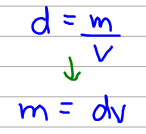Chemistry Practice Problems Density Practice Problems Solution: Mercury poisoning is a debilitating disease that i...

🤓 Based on our data, we think this question is relevant for Professor Gillespie's class at FIU.

# Solution: Mercury poisoning is a debilitating disease that is often fatal. In the human body, mercury reacts with essential enzymes leading to irreversible inactivity of these enzymes. If the amount of mercury in a polluted lake is 0.4 μg Hg/mL, what is the total mass in kilograms of mercury in the lake? (The lake has a surface area of 100 mi2 and an average depth of 20 ft.)

###### Problem

Mercury poisoning is a debilitating disease that is often fatal. In the human body, mercury reacts with essential enzymes leading to irreversible inactivity of these enzymes. If the amount of mercury in a polluted lake is 0.4 μg Hg/mL, what is the total mass in kilograms of mercury in the lake? (The lake has a surface area of 100 mi2 and an average depth of 20 ft.)

###### Solution

The density of Hg was given as 0.4 μg Hg/mL. Now the density is basically mass per unit volume so we can calculate mass using the density and the volume.However, we don't have a value for volume so we have to calculate that using the surface area and the depth using the formula below:View Complete Written Solution

Density

Density

#### Q. In each of the following pairs, which has the greater mass? (See Table R‑5.)d. 75 mL of copper or 1.0 L of benzene

Solved • Wed Oct 17 2018 11:10:09 GMT-0400 (EDT)

Density

#### Q. In each of the following pairs, which has the greater mass? (See Table R‑5.)c. 19.3 mL of water or 1.00 mL of gold

Solved • Wed Oct 17 2018 11:09:16 GMT-0400 (EDT)

Density

#### Q. For a material to float on the surface of water, the material must have a density less than that of water (1.0 g/mL) andmust not react with the water ...

Solved • Wed Oct 17 2018 10:46:46 GMT-0400 (EDT)

Density

#### Q. A large piece of jewelry has a mass of 132.6 g. A graduated cylinder initially contains 48.6 mL water. When the jewelry is submerged in the graduated ...

Solved • Tue Oct 16 2018 12:33:49 GMT-0400 (EDT)